# Fractions - 8th grade (13y) - examples - page 8

1. The farmer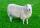The farmer had 140 sheep. For the next year, she decided to change the number of sheep in ratio 10: 7. How many sheep will he have then?
2. SeawaterSeawater has a density of 1025 kg/m3, ice 920 kg/m3. 8 liters of seawater froze and created a cube. Calculate the size of the cube edge.
3. Circular arcCalculate the length of the circular arc if the diameter d = 20cm and the angle alpha = 142 °
4. Three 43Three brothers inherited a cash amount of 62,000 and they divided it among themselves in the ratio of 5:4:1. How much more is the largest share than the smallest share?
5. Water reservoir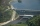One drain hole emptied the water from the tank for 20 hours, with the second drain for 30 hours. How long will the water drain from this tank if we open two drains simultaneously?
6. Red diplomasHe numbers of students with honors in 2013 and 2014 are in ratio 40:49. How big is the year-on-year percentage increase?
7. The sidesThe sides of a rectangle are in a ratio of 2:3, and its perimeter is 1 1/4 inches. What are the lengths of its side? Draw it.
8. Sewing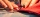Beth's mother can sew 235 pairs of short pants in 6 days while Lourdes can sew 187 pairs in 8 days. How many more pairs of short pants can Beth's mother sew?
9. New ratio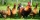The ratio of ducks and chicken in our yard is 2 : 3. The total number of ducks and chickens together is 30. Mother gave 3 of the chickens to our neighbor. What is the new ratio now?
10. Wiring 2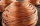Willie cut a piece of wire that was 3/8 of the total length of the wire. He cut another piece that was 9m long. The two pieces together were one half of the total length of the wire. How long was the wire before he cut it?
11. Equation - inverseSolve for x: 7: x = 14: 1000
12. Soup from canteen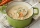For how many people is 90 liters of soup enough if we assume 3/8 liter of soup per person in the canteen?
13. Marriage sttusIn our city, there are 3/5 of the women married to 2/3 of the men. Find what part of the population is free.
14. Three segments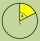The circle is divided into 3 segments. Segment A occupies 1/4 of the area, segment B occupies 1/3 of the area. What part is occupied by section C? In what proportion are areas A: B: C?
15. On a farm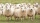On a farm, there are 90 rabbits, 700 sheep, 300 cattle and 500 pigs. What percentage of the total number of animals are rabbits?
16. Iron pole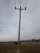The iron pole is in the ground 2/5 of its length, partly above the ground 1/3 is yellow, and the unpainted section is 6 m long. How long is the entire column?
17. PlayingHow long have we trained on the pitch when we know that the warm-up took 10 minutes, we trained passes for one-third of the time and we played football half the time?
18. Square metal sheetFour squares of 300 mm side were cut out from a square sheet metal plate with a side of 0,7 m. Express the fraction and the percentage of waste from the square metal sheet.
19. Castle towerThe castle tower has a cone-shaped roof with a diameter of 10 meters and a height of 8 meters. Calculate how much m² of coverage is needed to cover it if we must add one-third for the overlap.
20. Oranges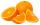Mother divided her three children's oranges in a ratio of 6:5:4. Two children gave 45 oranges. How many oranges were there?

Do you have an interesting mathematical example that you can't solve it? Enter it, and we can try to solve it.

To this e-mail address, we will reply solution; solved examples are also published here. Please enter e-mail correctly and check whether you don't have a full mailbox.

Need help calculate sum, simplify or multiply fractions? Try our fraction calculator.